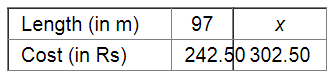# The cost of 97 metre of cloth is Rs 242.50.`
Question:

The cost of 97 metre of cloth is Rs 242.50. What length of this can be purchased for Rs 302.50?

Solution:

Let x metre be the length of the cloth that can be purchased for Rs 302.50.Since the length of the cloth and its cost are in direct variation, we have :

$\frac{97}{x}=\frac{242.50}{302.50}$

$\Rightarrow 97 \times 302.50=x \times 242.50$

$\Rightarrow x=\frac{97 \times 302.50}{242.50}$

$=\frac{29342.50}{242.50}$

$=121$

Thus, the required length will be 121 metre.